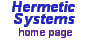# Prime Factors## 1. Introduction

Prime Factors may be used to:
• get the prime decomposition of all numbers in a range of numbers up to 2,147,483,646,
• get all factors (prime and non-prime) of a single number,
• get all factors of all numbers in a range of numbers,
• find only the prime numbers in a range of numbers,
• find pairs of prime numbers (e.g. 107 and 109) in a certain range,
• find Fermat primes and Mersenne primes < 2,147,483,647,
• draw the Ulam prime number spiral
• and more.
In addition a "filter" condition may be set to allow examination of numbers with a constant offset from an arithmetical or a geometrical progression, e.g., just those numbers of the form 7*n - 12 or 10n + 2. The main screen looks like this:Examples of the use of the program are given in the user manual. Following accepted mathematical convention, the number 1 is not held to be a prime number.